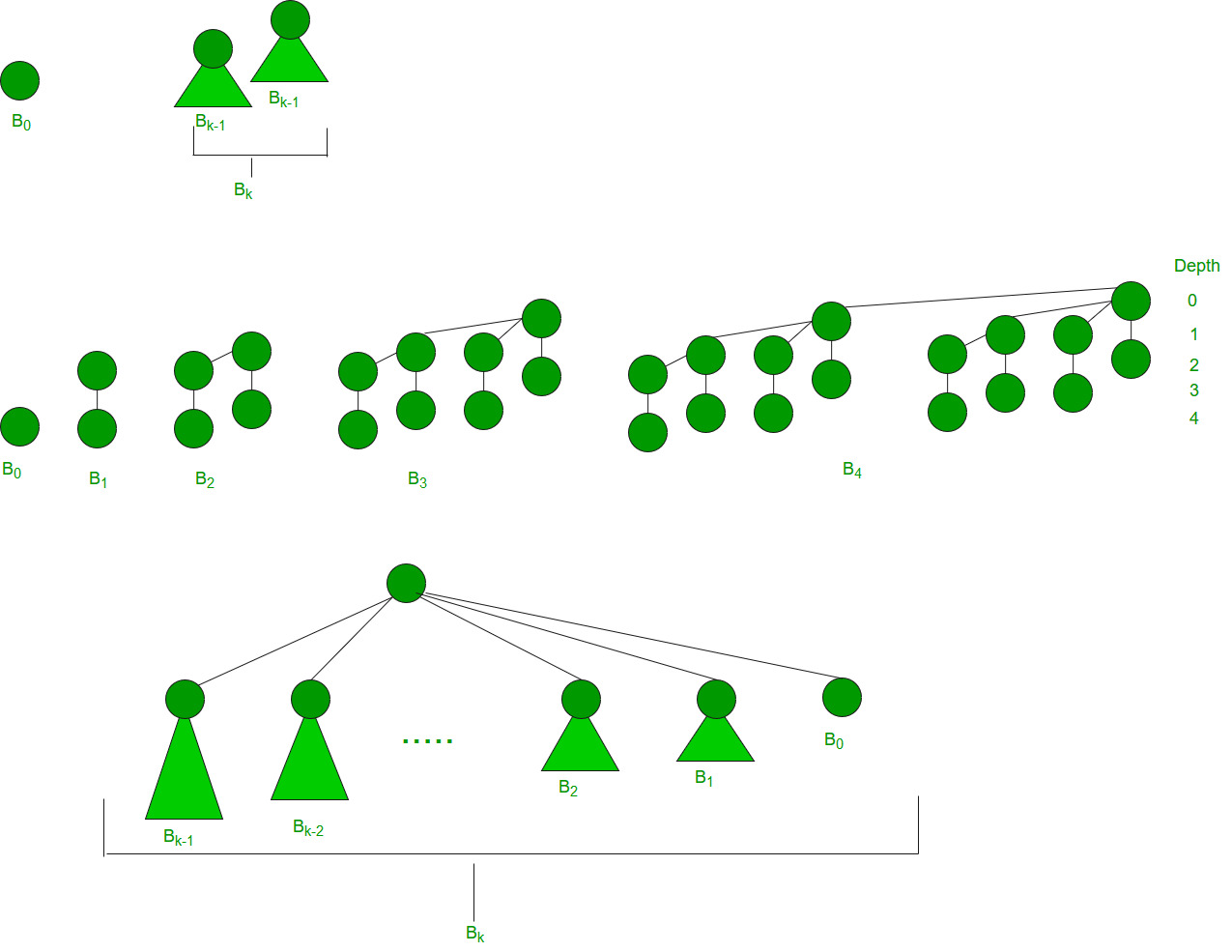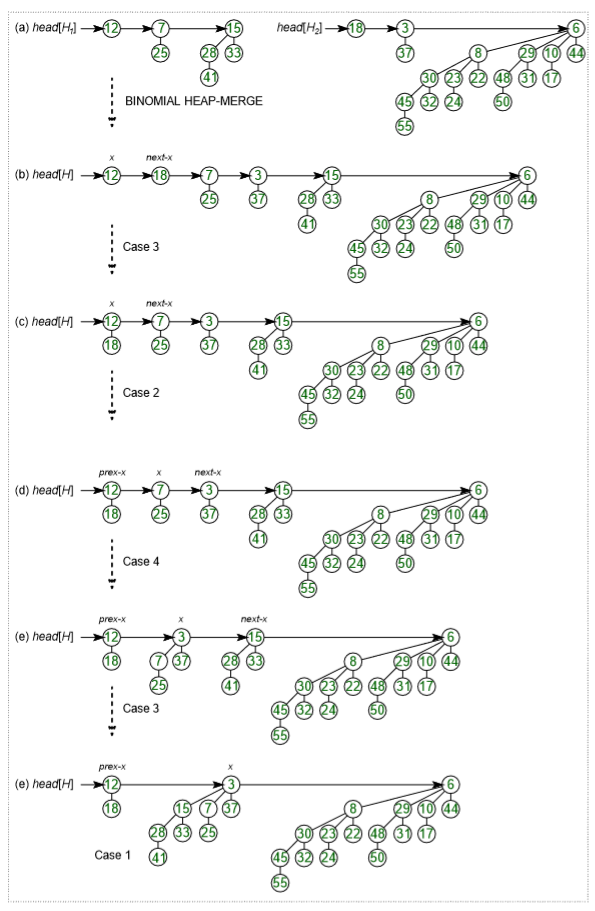# Binomial Heap

• Difficulty Level : Hard
• Last Updated : 04 Nov, 2020

The main application of Binary Heap is as implement priority queue. Binomial Heap is an extension of Binary Heap that provides faster union or merge operation together with other operations provided by Binary Heap.

A Binomial Heap is a collection of Binomial Trees

What is a Binomial Tree?
A Binomial Tree of order 0 has 1 node. A Binomial Tree of order k can be constructed by taking two binomial trees of order k-1 and making one as leftmost child or other.
A Binomial Tree of order k has following properties.
a) It has exactly 2k nodes.
b) It has depth as k.
c) There are exactly kCi nodes at depth i for i = 0, 1, . . . , k.
d) The root has degree k and children of root are themselves Binomial Trees with order k-1, k-2,.. 0 from left to right.

```k = 0 (Single Node)

o

k = 1 (2 nodes)
[We take two k = 0 order Binomial Trees, and
make one as child of other]
o
/
o

k = 2 (4 nodes)
[We take two k = 1 order Binomial Trees, and
make one as child of other]
o
/   \
o     o
/
o

k = 3 (8 nodes)
[We take two k = 2 order Binomial Trees, and
make one as child of other]
o
/  | \
o   o  o
/ \  |
o   o o
\
o
```

The following diagram is referred from 2nd Edition of CLRS bookBinomial Heap:
A Binomial Heap is a set of Binomial Trees where each Binomial Tree follows Min Heap property. And there can be at most one Binomial Tree of any degree.

Examples Binomial Heap:

```12------------10--------------------20
/  \                 /  | \
15    50             70  50  40
|                  / |    |
30               80  85  65
|
100
A Binomial Heap with 13 nodes. It is a collection of 3
Binomial Trees of orders 0, 2 and 3 from left to right.

10--------------------20
/  \                 /  | \
15    50             70  50  40
|                  / |    |
30               80  85  65
|
100
```

A Binomial Heap with 12 nodes. It is a collection of 2
Binomial Trees of orders 2 and 3 from left to right.

Binary Representation of a number and Binomial Heaps
A Binomial Heap with n nodes has the number of Binomial Trees equal to the number of set bits in the Binary representation of n. For example let n be 13, there 3 set bits in the binary representation of n (00001101), hence 3 Binomial Trees. We can also relate the degree of these Binomial Trees with positions of set bits. With this relation, we can conclude that there are O(Logn) Binomial Trees in a Binomial Heap with ‘n’ nodes.

Operations of Binomial Heap:
The main operation in Binomial Heap is union(), all other operations mainly use this operation. The union() operation is to combine two Binomial Heaps into one. Let us first discuss other operations, we will discuss union later.

1. insert(H, k): Inserts a key ‘k’ to Binomial Heap ‘H’. This operation first creates a Binomial Heap with single key ‘k’, then calls union on H and the new Binomial heap.
2. getMin(H): A simple way to getMin() is to traverse the list of root of Binomial Trees and return the minimum key. This implementation requires O(Logn) time. It can be optimized to O(1) by maintaining a pointer to minimum key root.
3. extractMin(H): This operation also uses union(). We first call getMin() to find the minimum key Binomial Tree, then we remove the node and create a new Binomial Heap by connecting all subtrees of the removed minimum node. Finally, we call union() on H and the newly created Binomial Heap. This operation requires O(Logn) time.
4. delete(H): Like Binary Heap, delete operation first reduces the key to minus infinite, then calls extractMin().
5. decreaseKey(H): decreaseKey() is also similar to Binary Heap. We compare the decreases key with it parent and if parent’s key is more, we swap keys and recur for the parent. We stop when we either reach a node whose parent has a smaller key or we hit the root node. Time complexity of decreaseKey() is O(Logn).

Union operation in Binomial Heap:
Given two Binomial Heaps H1 and H2, union(H1, H2) creates a single Binomial Heap.

6. The first step is to simply merge the two Heaps in non-decreasing order of degrees. In the following diagram, figure(b) shows the result after merging.
7. After the simple merge, we need to make sure that there is at most one Binomial Tree of any order. To do this, we need to combine Binomial Trees of the same order. We traverse the list of merged roots, we keep track of three-pointers, prev, x and next-x. There can be following 4 cases when we traverse the list of roots.
—–Case 1: Orders of x and next-x are not same, we simply move ahead.
In following 3 cases orders of x and next-x are same.
—–Case 2: If the order of next-next-x is also same, move ahead.
—–Case 3: If the key of x is smaller than or equal to the key of next-x, then make next-x as a child of x by linking it with x.
—–Case 4: If the key of x is greater, then make x as the child of next.

The following diagram is taken from 2nd Edition of CLRS bookHow to represent Binomial Heap?
A Binomial Heap is a set of Binomial Trees. A Binomial Tree must be represented in a way that allows sequential access to all siblings, starting from the leftmost sibling (We need this in and extractMin() and delete()). The idea is to represent Binomial Trees as the leftmost child and right-sibling representation, i.e., every node stores two pointers, one to the leftmost child and other to the right sibling.